# histogram

Plot histogram of multiparametric global sensitivity analysis results

## Syntax

``h = histogram(mpgsaObj)``
``h = histogram(mpgsaObj,Name,Value)``

## Description

example

````h = histogram(mpgsaObj)` plots a histogram of multiparametric global sensitivity analysis (MPGSA) results and returns the figure handle `h`.```

example

````h = histogram(mpgsaObj,Name,Value)` uses additional options specified by one or more name-value pair arguments.```

## Examples

collapse all

`sbioloadproject tmdd_with_TO.sbproj`

Get the active configset and set the target occupancy (`TO`) as the response.

```cs = getconfigset(m1); cs.RuntimeOptions.StatesToLog = 'TO';```

Simulate the model and plot the `TO` profile.

`sbioplot(sbiosimulate(m1,cs));`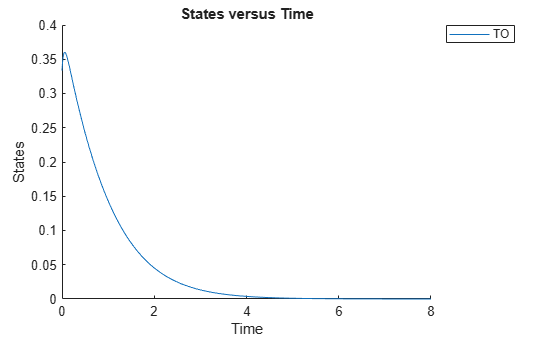Define an exposure (area under the curve of the TO profile) threshold for the target occupancy.

`classifier = 'trapz(time,TO) <= 0.1';`

Perform MPGSA to find sensitive parameters with respect to the TO. Vary the parameter values between predefined bounds to generate 10,000 parameter samples.

```% Suppress an information warning that is issued during simulation. warnSettings = warning('off', 'SimBiology:sbservices:SB_DIMANALYSISNOTDONE_MATLABFCN_UCON'); rng(0,'twister'); % For reproducibility params = {'kel','ksyn','kdeg','km'}; bounds = [0.1, 1; 0.1, 1; 0.1, 1; 0.1, 1]; mpgsaResults = sbiompgsa(m1,params,classifier,'Bounds',bounds,'NumberSamples',10000)```
```mpgsaResults = MPGSA with properties: Classifiers: {'trapz(time,TO) <= 0.1'} KolmogorovSmirnovStatistics: [4x1 table] ECDFData: {4x4 cell} SignificanceLevel: 0.0500 PValues: [4x1 table] SupportHypothesis: [10000x1 table] ParameterSamples: [10000x4 table] Observables: {'TO'} SimulationInfo: [1x1 struct] ```

Plot the quantiles of the simulated model response.

`plotData(mpgsaResults);`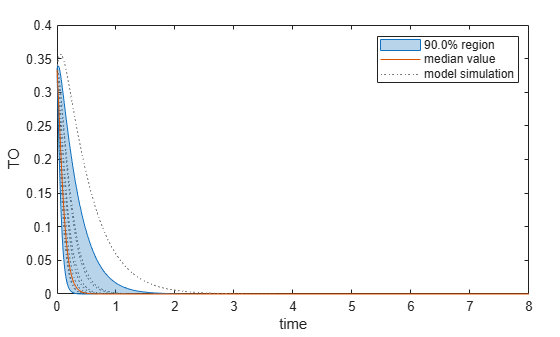Plot the empirical cumulative distribution functions (eCDFs) of the accepted and rejected samples. Except for `km`, none of the parameters shows a significant difference in the eCDFs for the accepted and rejected samples. The `km` plot shows a large Kolmogorov-Smirnov (K-S) distance between the eCDFs of the accepted and rejected samples. The K-S distance is the maximum absolute distance between two eCDFs curves.

```h = plot(mpgsaResults); % Resize the figure. pos = h.Position(:); h.Position(:) = [pos(1) pos(2) pos(3)*2 pos(4)*2];```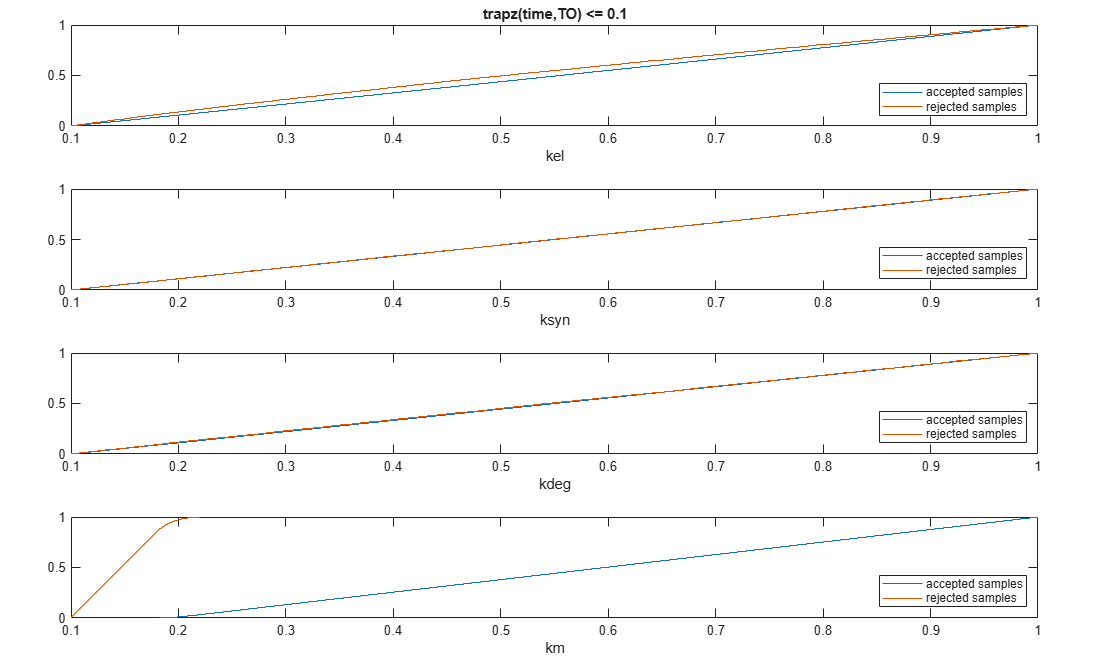To compute the K-S distance between the two eCDFs, SimBiology uses a two-sided test based on the null hypothesis that the two distributions of accepted and rejected samples are equal. See `kstest2` (Statistics and Machine Learning Toolbox) for details. If the K-S distance is large, then the two distributions are different, meaning that the classification of the samples is sensitive to variations in the input parameter. On the other hand, if the K-S distance is small, then variations in the input parameter do not affect the classification of samples. The results suggest that the classification is insensitive to the input parameter. To assess the significance of the K-S statistic rejecting the null-hypothesis, you can examine the p-values.

`bar(mpgsaResults)`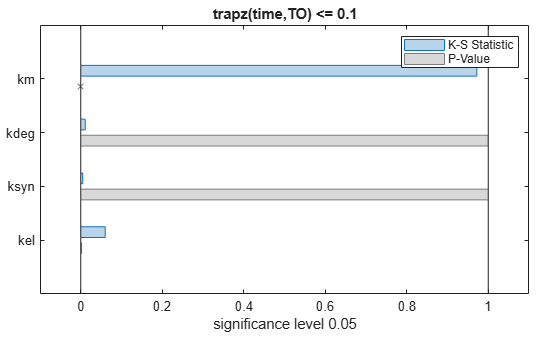The bar plot shows two bars for each parameter: one for the K-S distance (K-S statistic) and another for the corresponding p-value. You reject the null hypothesis if the p-value is less than the significance level. A cross (`x`) is shown for any p-value that is almost 0. You can see the exact p-value corresponding to each parameter.

`[mpgsaResults.ParameterSamples.Properties.VariableNames',mpgsaResults.PValues]`
```ans=4×2 table Var1 trapz(time,TO) <= 0.1 ________ _____________________ {'kel' } 0.0021877 {'ksyn'} 1 {'kdeg'} 0.99983 {'km' } 0 ```

The p-values of `km` and `kel` are less than the significance level (0.05), supporting the alternative hypothesis that the accepted and rejected samples come from different distributions. In other words, the classification of the samples is sensitive to `km` and `kel` but not to other parameters (`kdeg` and `ksyn`).

You can also plot the histograms of accepted and rejected samples. The historgrams let you see trends in the accepted and rejected samples. In this example, the histogram of `km` shows that there are more accepted samples for larger `km` values, while the `kel` histogram shows that there are fewer rejected samples as `kel` increases.

```h2 = histogram(mpgsaResults); % Resize the figure. pos = h2.Position(:); h2.Position(:) = [pos(1) pos(2) pos(3)*2 pos(4)*2];```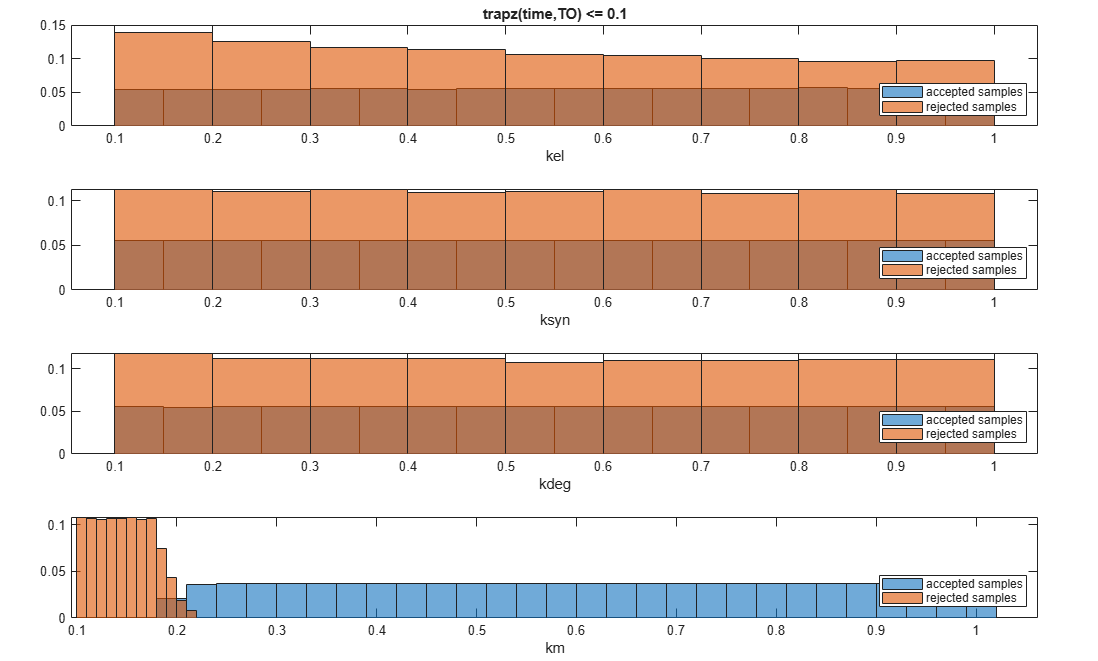Restore the warning settings.

`warning(warnSettings);`

## Input Arguments

collapse all

Multiparametric global sensitivity analysis results, specified as a `SimBiology.gsa.MPGSA` object.

### Name-Value Arguments

Specify optional comma-separated pairs of `Name,Value` arguments. `Name` is the argument name and `Value` is the corresponding value. `Name` must appear inside quotes. You can specify several name and value pair arguments in any order as `Name1,Value1,...,NameN,ValueN`.

Example: `h = histogram(results,'Classifier',1)` specifies to plot histograms of MPGSA results of the first classifier.

Input model quantities, namely parameters, species, or compartments, to plot, specified as the comma-separated pair consisting of `'Parameters'` and a character vector, string, string vector, cell array of character vectors, or a vector of positive integers indexing into the columns of the `mpgsaObj.ParameterSamples` table.

Example: `'Parameters','k1'`

Data Types: `double` | `char` | `string` | `cell`

Classifiers to plot, specified as the comma-separated pair consisting of `'Classifiers'` and a character vector, string, string vector, cell array of character vectors, or a vector of positive integers.

Specify the expressions of classifiers to plot as a character vector, string, string vector, cell array of character vectors. Alternatively, you can specify a vector of positive integers indexing into `mpgsaObj.Classifiers`.

Example: `'Classifiers',[1 3]`

Data Types: `double` | `char` | `string` | `cell`

Color of eCDFs of accepted samples, specified as the comma-separated pair consisting of `'AcceptedSamplesColor'` and a three-element row vector. By default, the function uses the first MATLAB® default color. To view the default color order, enter `get(groot,'defaultAxesColorOrder')` or see the ColorOrder property.

Example: `'AcceptedSamplesColor',[0.4,0.3,0.2]`

Data Types: `double`

Color of eCDFs of rejected samples, specified as the comma-separated pair consisting of `'RejectedSamplesColor'` and a three-element row vector. By default, the function uses the second MATLAB default color for the first order and the second default color for the total order. To view the default color order, enter `get(groot,'defaultAxesColorOrder')` or see the ColorOrder property.

Example: `'RejectedSamplesColor',[0.9,0.5,0.2]`

Data Types: `double`

## Output Arguments

collapse all

Handle to the figure, specified as a figure handle.

 Tiemann, Christian A., Joep Vanlier, Maaike H. Oosterveer, Albert K. Groen, Peter A. J. Hilbers, and Natal A. W. van Riel. “Parameter Trajectory Analysis to Identify Treatment Effects of Pharmacological Interventions.” Edited by Scott Markel. PLoS Computational Biology 9, no. 8 (August 1, 2013): e1003166. https://doi.org/10.1371/journal.pcbi.1003166.

## Support평가판 신청